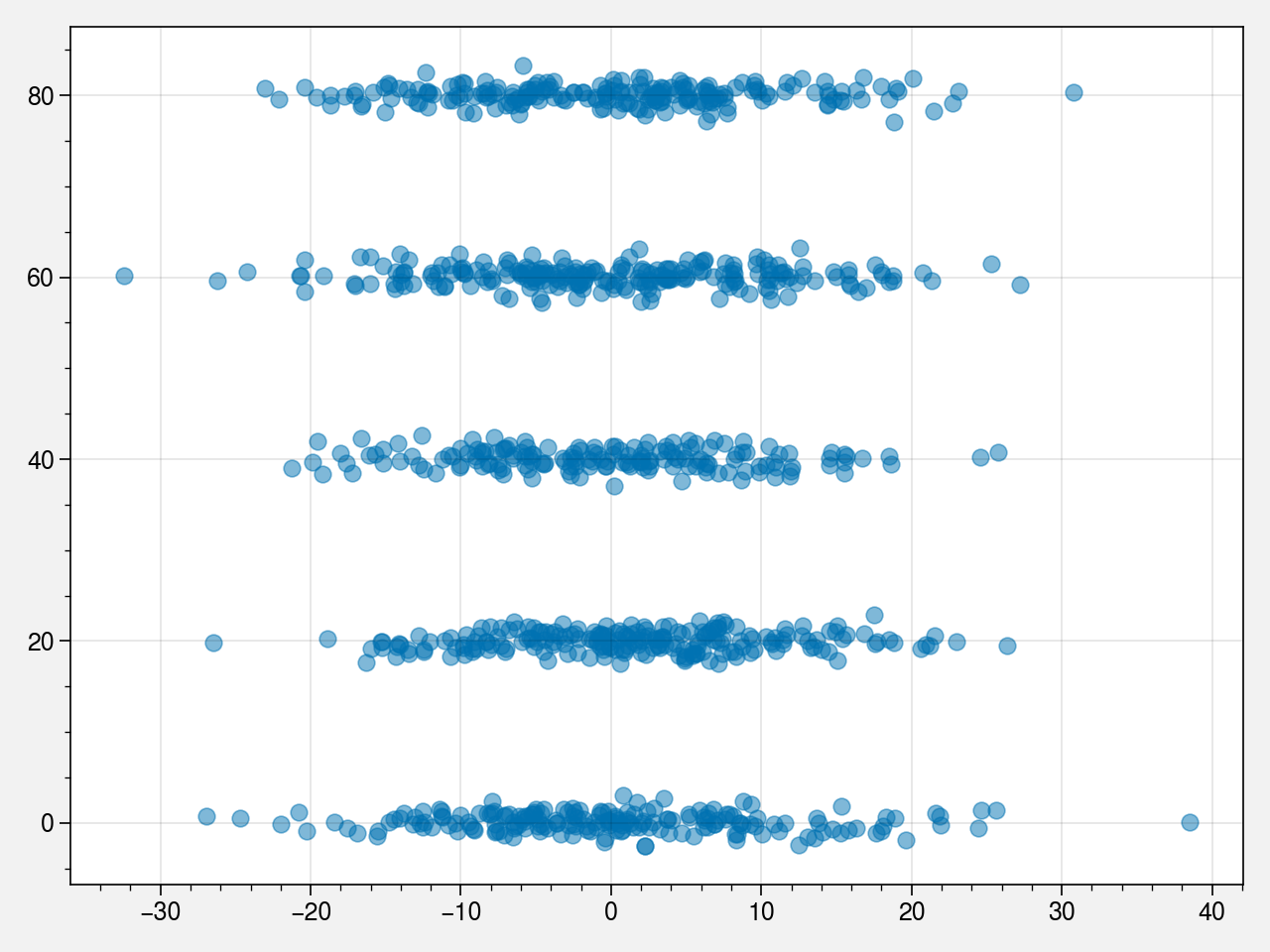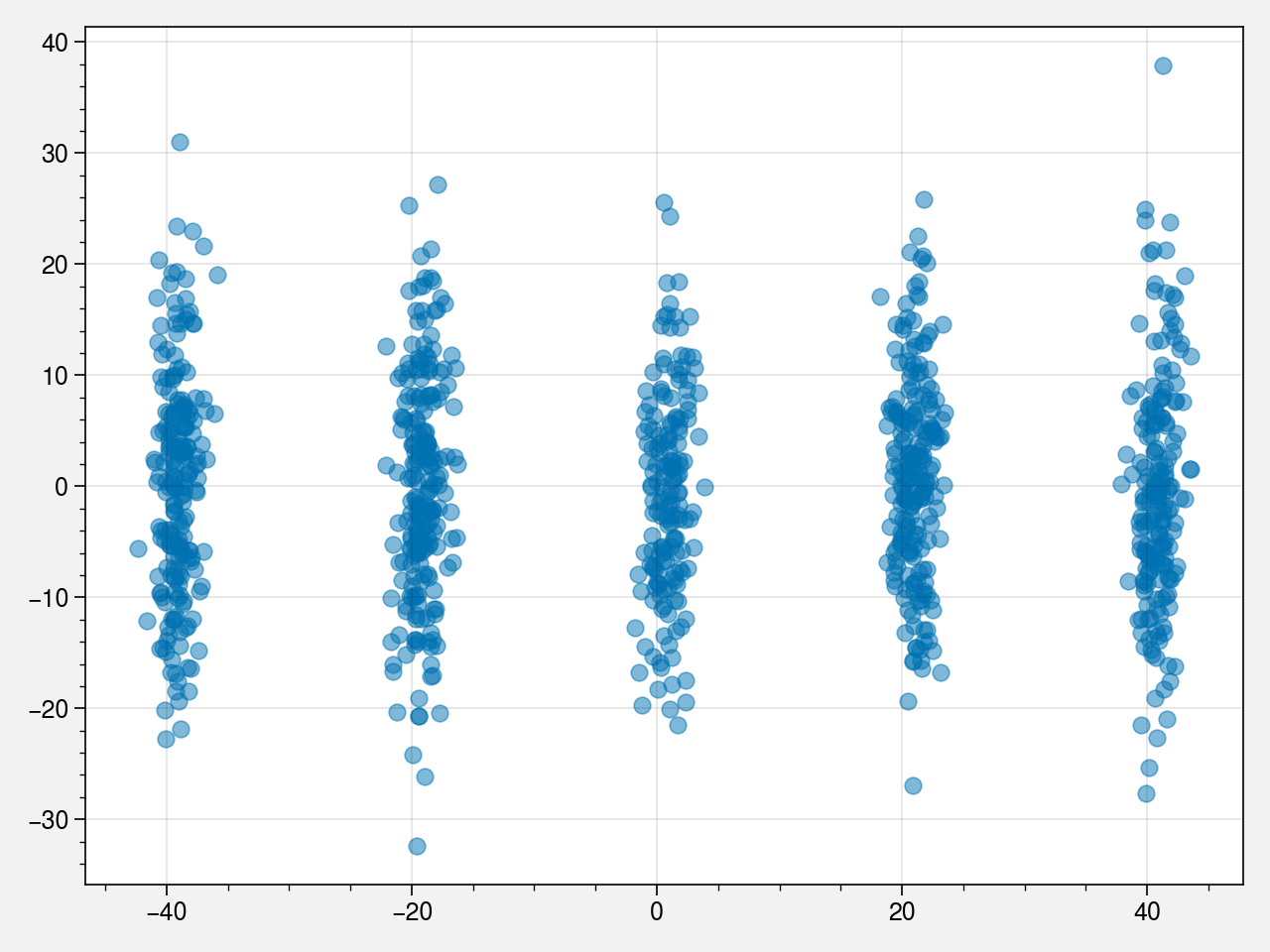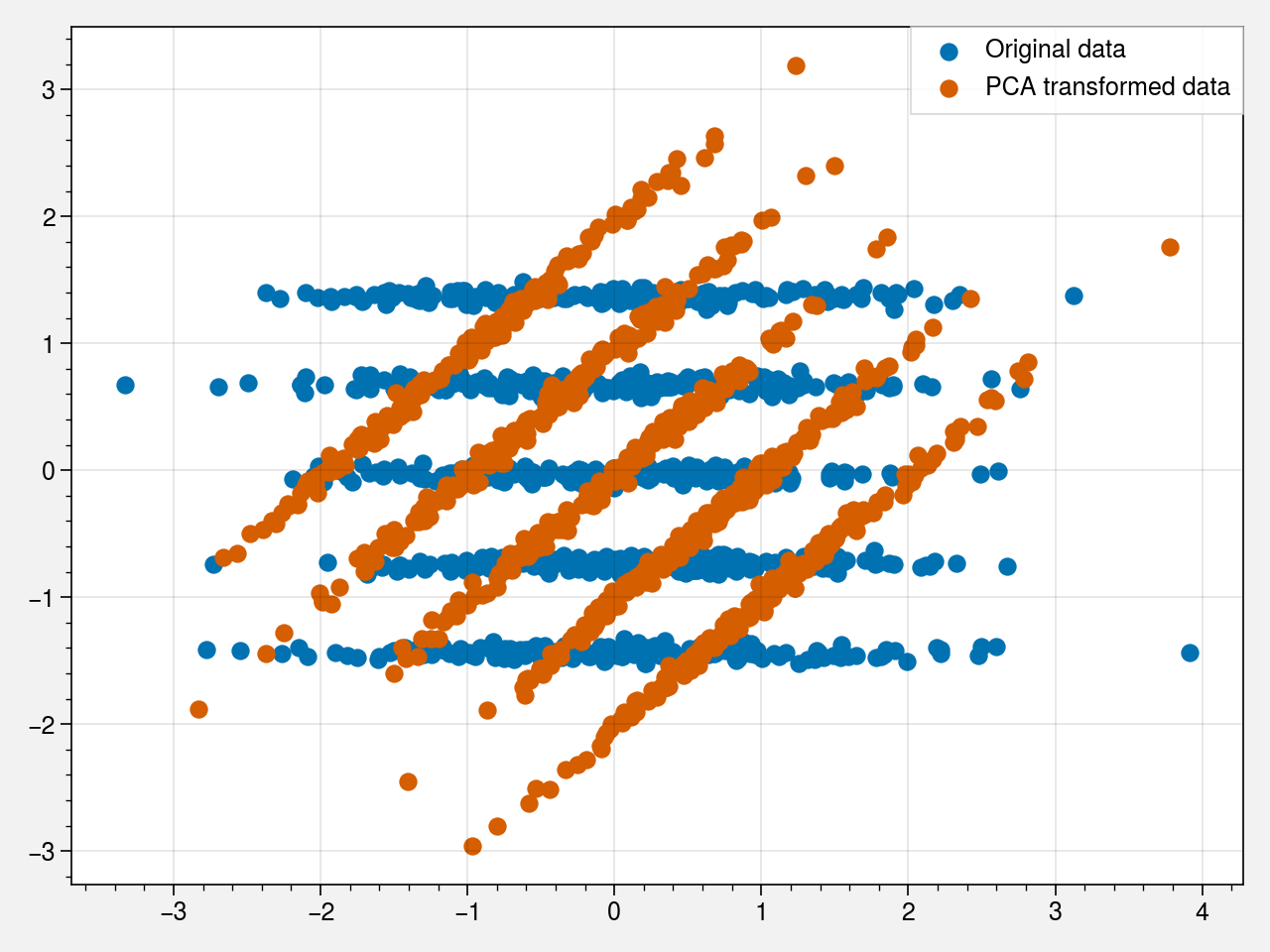# Why does this PCA have stripes?¶

PCA is often used as a dimensionality reduction tool to visualize the structure in your data. Given a dataset with multiple dimensions (features), PCA finds a reduced dimension representation. In the simplest case of two dimensions, you can think of PCA as a mere rotation of your Y and X axis such that the rotated first axis (PC1) represents the dimension along which you have maximum variation. The principal components are so numbered that the amount of variation in a particular direction reduces as the PC number increases. In short, $PC1>PC2>PC3\dots > PCn$ in terms of the variance expained.

The figure from the tweet above asks a very simple question: why do the points form a strip like pattern? Often in such analysis questions, we are interested in blobs which are often take to represent "clusters". We all would be happy if for example, all the shapes (+,x,o,square) resulted in a blob or if the points appeared in colored blobs of unique colors. I do not have much information about the metadata associated with each point but each color/symbol combo is a sample location.

In :
#hide
!pip install proplot

Requirement already satisfied: proplot in /usr/local/lib/python3.6/dist-packages (0.6.4)
Requirement already satisfied: matplotlib in /usr/local/lib/python3.6/dist-packages (from proplot) (3.2.2)
Requirement already satisfied: cycler>=0.10 in /usr/local/lib/python3.6/dist-packages (from matplotlib->proplot) (0.10.0)
Requirement already satisfied: numpy>=1.11 in /usr/local/lib/python3.6/dist-packages (from matplotlib->proplot) (1.18.5)
Requirement already satisfied: kiwisolver>=1.0.1 in /usr/local/lib/python3.6/dist-packages (from matplotlib->proplot) (1.2.0)
Requirement already satisfied: python-dateutil>=2.1 in /usr/local/lib/python3.6/dist-packages (from matplotlib->proplot) (2.8.1)
Requirement already satisfied: pyparsing!=2.0.4,!=2.1.2,!=2.1.6,>=2.0.1 in /usr/local/lib/python3.6/dist-packages (from matplotlib->proplot) (2.4.7)
Requirement already satisfied: six in /usr/local/lib/python3.6/dist-packages (from cycler>=0.10->matplotlib->proplot) (1.12.0)

In :
#hide
%pylab inline
import proplot
from sklearn.decomposition import PCA
np.random.seed(42)

Populating the interactive namespace from numpy and matplotlib

In :
N = 1000

In :
# x-axis is normally distributed
x = np.random.normal(0, 10, N)
# y-axis has observations that are identically distributed
# with a class structure + some noise
y = np.random.randint(0, 5, N) * 20 + np.random.normal(0, 1, N)
X = np.vstack((x,y)).T


If you start with a very simple dataset that has some "structure" it is possible to see such stripes in your data. Here I started off with a simple dataset that just has two dimensions. Along the x-axis the observations follow a gaussian distribution, while along the y axis we have some "structure". Observations in their y axis can belong to ,say 5, different modes. In short, the dataset looks like this

In :
#hide
plt.scatter(X[:,0], X[:,1], alpha=0.5)
plt.tight_layout()What would the PCA look like? The maximum amonut of variation in this case arises because of the data having multiple modes and as such the PCA has the following form (note that I did not scale the values to have zeor mean and unit variance) :

In :
# pca
fit = PCA(n_components=2).fit(X)
X_transformed = fit.transform(X)
plt.scatter(X_transformed[:,0], X_transformed[:,1], alpha=0.5)
plt.tight_layout()The stripes in the original tweet is essentially a derivative of such a case. The points along each stripe could have originated from a certain "batch" representing a mode. It is difficult to comment on what this "batch: could be without knowing a bit more about the data itself.

The above PCA plot worked on original X values. In this case, if you really think about how I generated the data, the actual scales of x and y in the original dataset do not have any meaning. If I were to treat them mutually exchangable, I could have worked with a 'standardized' or 'scaled' version of them (zero mean, unit variance), but the stripes would remain so.

In :
# hide
from sklearn.preprocessing import scale
X = np.vstack((x,y)).T
X_scaled = scale(X)

fit = PCA(n_components=2).fit(X_scaled)
X_transformed = fit.transform(X_scaled)
plt.scatter(X_scaled[:,0], X_scaled[:,1], label='Original data')
plt.scatter(X_transformed[:,0], X_transformed[:,1], label='PCA transformed data')
plt.legend()
plt.tight_layout()PCA is not the only choice of dimensionlity reduction. There are at least dozens of methods out there to do so. The choice is often guided by what we are really looking for and what we really know about the data.

Here's a figure I drew to highlight PCA with ICA and NMF, two other populer and related dimensionality reduction techniques.